# Hensel lemma

(diff) ← Older revision | Latest revision (diff) | Newer revision → (diff)

A statement obtained by K. Hensel  in the creation of the theory of-adic numbers (cf.-adic number), which subsequently found extensive use in commutative algebra. One says that Hensel's lemma is valid for a local ringwith maximal ideal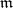if for any unitary polynomial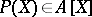and decompositionof its reduction modulointo a product of two mutually-prime polynomials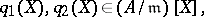there exist polynomials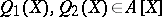such that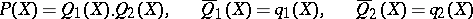(here the bar denotes the image under the reduction). In particular, for any simple rootof the reduced polynomial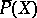there exists a solution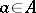of the equation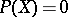which satisfies the condition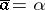. Hensel's lemma is fulfilled, for example, for a complete local ring. Hensel's lemma makes it possible to reduce the solution of an algebraic equation over a complete local ring to the solution of the corresponding equation over its residue field. Thus, in the ring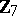of-adic numbers, Hensel's lemma yields the solvability of the equation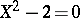, since this equation has two simple roots in the field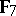of seven elements. A local ring for which Hensel's lemma is valid is known as a Hensel ring.

For Hensel's lemma in the non-commutative case see .

How to Cite This Entry:
Hensel lemma. Encyclopedia of Mathematics. URL: http://encyclopediaofmath.org/index.php?title=Hensel_lemma&oldid=15048
This article was adapted from an original article by V.I. Danilov (originator), which appeared in Encyclopedia of Mathematics - ISBN 1402006098. See original article